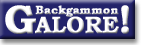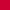TheoryForum Archive : Theory

 Janowski's formulas

 From: Stig Eide Address: stigeide@yahoo.com Date: 2 September 1999 Subject: Converting cubeless to cubefull Forum: rec.games.backgammon Google: 7qlqvg\$cmg\$1@nnrp1.deja.com

```I'd like to start a discussion of how to convert a cubeless equity-
estimate to cubeful. I guess the easiest is to start with moneygame,
and then generalize to match later.
I suspect that one way to go is to use the Janowski method. That's a
set of formulas that gives the needed win% to take and be too good.
I'll start by describing the formulas, using an example:
Let's say the player on roll has these probabilities (cubeless):

Player            Opponent
------------      -------------
BG    G    W      W     G    BG
0   30   60     40     10    0

That is, the player wins 60%, including 30% gammons. While he loses
40%, including 10% gammons. No bg's in this example. The cubeless
equity for Player (for the rest of the article I'll refer to 'Player'
as the player on roll and 'Opponent' as the other) is +0.4. The
cubefull equity will depend upon the position of the cube and its
value. I'll assume that the cube always has the value '1'. If Player
owns the cube, the equity should be higher than if Opponent owns it.
And if it's in the middle, the equity should be somewhere in between.

The Janowski formulaes uses the expected points won, for both players,
_given_ they win. That is, if Player win he'll win gammon in 30 of the
60%, and normal in 30 of the 60%. That is an average of 1.5 points pr.
won game. (60+30)/60 would also do the trick. Opponent will win 1.25
points pr. won game. These figures are cubeless. If we name expected
points for Player EP and expected points for Opponent EO, The Janowski
takepoint for Opponent, OT, is:

EP - 0.5
OT = -------------
EP + EO + c/2

'c' is cube-efficiency, a number between 0 and 1. That is, if the cube
is dead, c will be 0. In the continuous model, c is 1 (all doubles are
borderline take/pass). This number will depend upon the position. From
now on I'll just estimate it to be 3/4. It's more common to estimate it
to be 2/3 but that's another discussion. If we use the example, we find
that Opponents takepoint is 32%. That is, to take a double he need to
win 32% cubeless when he factor in gammons and the value of owning the
cube. Note that Player would have a cubefull equity of 1, if he had had
68% wins, because Opponent would then have a borderline take/pass. We
also need Players takepoint. I'll assume that the expected points won
for Player and Opponent is the best estimate for this position and all
of the future ones. This is a crucial assumption. I'm not sure if it is
sound to assume that Players gammonrate will be independent of his
wins. But, using this assumption, the Janowski takepoint for player,
PT, is:

EO - 0.5
PT = -------------
EO + EP + c/2

The cubeless figures in the example and a cubeefficiency of 3/4, says
that Players takepoint is 24%. We should now be ready to calculate the
cubefull equities: If the cube is in the middle, Player can cash if he
reach 68% wins, while he has a drop if he reach 24%. He's now at 60%,
so the probability that he reach 68% before 24% should be:

60 - 24   36
------- = -- = 82%
68 - 24   44

So, Player will win one point in 82% and lose one point in the
remaining 18%. His cubefull equity is 1*0.82 + (-1)*0.18 = 0.64
If Player owns the cube, he can still cash at 68%, but he has the right
to play until he loses, so the probability of Player cashing before he
loses is:

60 - 0   60
------ = -- = 88%
68 - 0   68

So, player will win one point in 88% and lose 1.25 in the remaining
12%. His cubefull equity is 1*0.88 + (-1.25)*0.18 = 0.655
If Opponent owns the cube, Player needs to climb to 100% before he gets
his expected 1.5 points while Opponent gets one point if Player gets to
24%. The probability of this happening is:

60 - 24     36
-------- =  -- = 47%
100 - 24    76

So, Player will win 1.5 points in 47% and lose one point in the
remaining 53%. His cubefull equity is 1.5*0.47 + (-1)*0.53 = 0.175

The remaining part is to figure out cubefull equity when one of the
players are too good to double. The Janowski formula for the too good
point is in the same spirit as the formula for take point: Player is
too good if his probability of winnig is more than

EO - 1
-------------
EP + EO + c/2

Using the same method as earlier, we should be able to convert cubeless
equity to cubefull in positions where one of the players are too good.

Questions, anyone?
Stig Eide
```

### Theory

Derivation of drop points  (Michael J. Zehr, Apr 1998)
Double/take/drop rates  (Gary Wong, June 1999)
Drop rate on initial doubles  (Gary Wong, July 1998)
Error rate--Why count forced moves?  (Ian Shaw+, Apr 2009)
Error rates--Repeated ND errors  (Joe Russell+, July 2009)
Inconsistencies in how EMG equity is calculated  (Jeremy Bagai, Nov 2007)Janowski's formulas  (Joern Thyssen+, Aug 2000)
Janowski's formulas  (Stig Eide, Sept 1999)
Jump Model for money game cube decisions  (Mark Higgins+, Mar 2012)
Number of distinct positions  (Walter Trice, June 1997)
Number of no-contact positions  (Darse Billings+, Mar 2004)
Optimal strategy?  (Gary Wong, July 1998)
Proof that backgammon terminates  (Robert Koca+, May 1994)Solvability of backgammon  (Gary Wong, June 1998)
Undefined equity  (Paul Tanenbaum+, Aug 1997)Under-doubling dice  (Bill Taylor, Dec 1997)Variance reduction  (Oliver Riordan, July 2003)From GammOnLineLong messageRecommended readingRecent addition

 Book Suggestions Books Cheating Chouettes Computer Dice Cube Handling Cube Handling in Races Equipment Etiquette Extreme Gammon Fun and frustration GNU Backgammon History Jellyfish Learning Luck versus Skill Magazines & E-zines Match Archives Match Equities Match Play Match Play at 2-away/2-away Miscellaneous Opening Rolls Pip Counting Play Sites Probability and Statistics Programming Propositions Puzzles Ratings Rollouts Rules Rulings Snowie Software Source Code Strategy--Backgames Strategy--Bearing Off Strategy--Checker play Terminology Theory Tournaments Uncategorized Variations